# Prepositional Logic – Simple Statements

Prepositional Logic is kind of logic that studies “Statements” and derives relationship among those statements.

#### What is a statement or a preposition ?

When we talk, we make many sentences, but all sentences are not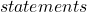. A sentence qualifies as a statement when it has a truth value. There is only two truth value for a statement –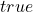or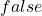.

For example,

What \hspace{5px}is \hspace{5px}your \hspace{5px}name?

The above sentence is a question, and we cannot give a truth value for this sentence. It is neither true nor false.

If someone reply to the question with an answer such as following

My \hspace{5px}name \hspace{5px} is \hspace{5px} Peter.

The above is an example of statement because it can be true or false.

### Simple Preposition and Compound Preposition

Suppose there are two prepositions.

\begin{aligned}
&I \hspace{5px}am \hspace{5px} eating.\\
&I \hspace{5px}am \hspace{5px} sleeping.
\end{aligned}

Such individual statement is called simple prepositions and we do not need to write them every time. Instead, we can assign an alphabet to each of these statements.

\begin{aligned}
&p : I \hspace{5px} am \hspace{5px} eating.\\
&q:  I\hspace{5px}  am\hspace{5px}  sleeping.
\end{aligned}

We can also make complex statements using these simple prepositions. However, we need a logical connective to do that.

There are many logical connectives but the most common connectives are

\begin{aligned}
&and \hspace{5px} ( conjunction )\\
&or  \hspace{5px}  ( disjunction )
\end{aligned}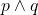and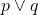are compound statements.

It means following

\begin{aligned}
&p \wedge q : I \hspace{5px} am \hspace{5px} eating \hspace{5px}and \hspace{5px}I \hspace{5px}am \hspace{5px}sleeping.\\
&p \vee q : I \hspace{5px}am \hspace{5px}eating \hspace{5px}or \hspace{5px}I \hspace{5px}am \hspace{5px}sleeping.
\end{aligned}

The truth value of the compound preposition depends on the individual simple preposition in the compound preposition.

In the above example, suppose truth value of p is true and q is false, then

\begin{aligned}
p \wedge q = true \wedge false = false
\end{aligned}

Similarly,

\begin{aligned}
p \vee q = true \vee false = true
\end{aligned}

We shall see more of these using a truth table in future lessons.